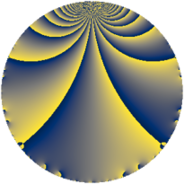Properties

 Label 6384.2.a.bpLevel $6384$ Weight $2$ Character orbit 6384.a Self dual yes Analytic conductor $50.976$ Analytic rank $1$ Dimension $2$ CM no Inner twists $1$

Related objects

Newspace parameters

 Level: $$N$$ $$=$$ $$6384 = 2^{4} \cdot 3 \cdot 7 \cdot 19$$ Weight: $$k$$ $$=$$ $$2$$ Character orbit: $$[\chi]$$ $$=$$ 6384.a (trivial)

Newform invariants

 Self dual: yes Analytic conductor: $$50.9764966504$$ Analytic rank: $$1$$ Dimension: $$2$$ Coefficient field: $$\Q(\sqrt{2})$$ Defining polynomial: $$x^{2} - 2$$ Coefficient ring: $$\Z[a_1, \ldots, a_{5}]$$ Coefficient ring index: $$1$$ Twist minimal: no (minimal twist has level 1596) Fricke sign: $$1$$ Sato-Tate group: $\mathrm{SU}(2)$

$q$-expansion

Coefficients of the $$q$$-expansion are expressed in terms of $$\beta = \sqrt{2}$$. We also show the integral $$q$$-expansion of the trace form.

 $$f(q)$$ $$=$$ $$q + q^{3} + \beta q^{5} - q^{7} + q^{9} +O(q^{10})$$ $$q + q^{3} + \beta q^{5} - q^{7} + q^{9} -2 q^{11} + 2 \beta q^{13} + \beta q^{15} -3 \beta q^{17} - q^{19} - q^{21} + ( -2 - 4 \beta ) q^{23} -3 q^{25} + q^{27} + ( 2 + \beta ) q^{29} + ( 2 - 2 \beta ) q^{31} -2 q^{33} -\beta q^{35} + ( 2 - 6 \beta ) q^{37} + 2 \beta q^{39} + ( 4 + 4 \beta ) q^{41} -2 q^{43} + \beta q^{45} + 5 \beta q^{47} + q^{49} -3 \beta q^{51} + ( 2 - 7 \beta ) q^{53} -2 \beta q^{55} - q^{57} + ( -8 + 4 \beta ) q^{59} + ( -2 - 2 \beta ) q^{61} - q^{63} + 4 q^{65} + ( -4 - 6 \beta ) q^{67} + ( -2 - 4 \beta ) q^{69} + ( -14 + \beta ) q^{71} + ( -6 + 4 \beta ) q^{73} -3 q^{75} + 2 q^{77} + ( -12 - 2 \beta ) q^{79} + q^{81} + \beta q^{83} -6 q^{85} + ( 2 + \beta ) q^{87} -4 q^{89} -2 \beta q^{91} + ( 2 - 2 \beta ) q^{93} -\beta q^{95} + ( 2 + 4 \beta ) q^{97} -2 q^{99} +O(q^{100})$$ $$\operatorname{Tr}(f)(q)$$ $$=$$ $$2 q + 2 q^{3} - 2 q^{7} + 2 q^{9} + O(q^{10})$$ $$2 q + 2 q^{3} - 2 q^{7} + 2 q^{9} - 4 q^{11} - 2 q^{19} - 2 q^{21} - 4 q^{23} - 6 q^{25} + 2 q^{27} + 4 q^{29} + 4 q^{31} - 4 q^{33} + 4 q^{37} + 8 q^{41} - 4 q^{43} + 2 q^{49} + 4 q^{53} - 2 q^{57} - 16 q^{59} - 4 q^{61} - 2 q^{63} + 8 q^{65} - 8 q^{67} - 4 q^{69} - 28 q^{71} - 12 q^{73} - 6 q^{75} + 4 q^{77} - 24 q^{79} + 2 q^{81} - 12 q^{85} + 4 q^{87} - 8 q^{89} + 4 q^{93} + 4 q^{97} - 4 q^{99} + O(q^{100})$$

Embeddings

For each embedding $$\iota_m$$ of the coefficient field, the values $$\iota_m(a_n)$$ are shown below.

For more information on an embedded modular form you can click on its label.

Label $$\iota_m(\nu)$$ $$a_{2}$$ $$a_{3}$$ $$a_{4}$$ $$a_{5}$$ $$a_{6}$$ $$a_{7}$$ $$a_{8}$$ $$a_{9}$$ $$a_{10}$$
1.1
 −1.41421 1.41421
0 1.00000 0 −1.41421 0 −1.00000 0 1.00000 0
1.2 0 1.00000 0 1.41421 0 −1.00000 0 1.00000 0
 $$n$$: e.g. 2-40 or 990-1000 Significant digits: Format: Complex embeddings Normalized embeddings Satake parameters Satake angles

Atkin-Lehner signs

$$p$$ Sign
$$2$$ $$-1$$
$$3$$ $$-1$$
$$7$$ $$1$$
$$19$$ $$1$$

Inner twists

This newform does not admit any (nontrivial) inner twists.

Twists

By twisting character orbit
Char Parity Ord Mult Type Twist Min Dim
1.a even 1 1 trivial 6384.2.a.bp 2
4.b odd 2 1 1596.2.a.h 2
12.b even 2 1 4788.2.a.h 2

By twisted newform orbit
Twist Min Dim Char Parity Ord Mult Type
1596.2.a.h 2 4.b odd 2 1
4788.2.a.h 2 12.b even 2 1
6384.2.a.bp 2 1.a even 1 1 trivial

Hecke kernels

This newform subspace can be constructed as the intersection of the kernels of the following linear operators acting on $$S_{2}^{\mathrm{new}}(\Gamma_0(6384))$$:

 $$T_{5}^{2} - 2$$ $$T_{11} + 2$$ $$T_{13}^{2} - 8$$ $$T_{17}^{2} - 18$$ $$T_{23}^{2} + 4 T_{23} - 28$$

Hecke characteristic polynomials

$p$ $F_p(T)$
$2$ $$T^{2}$$
$3$ $$( -1 + T )^{2}$$
$5$ $$-2 + T^{2}$$
$7$ $$( 1 + T )^{2}$$
$11$ $$( 2 + T )^{2}$$
$13$ $$-8 + T^{2}$$
$17$ $$-18 + T^{2}$$
$19$ $$( 1 + T )^{2}$$
$23$ $$-28 + 4 T + T^{2}$$
$29$ $$2 - 4 T + T^{2}$$
$31$ $$-4 - 4 T + T^{2}$$
$37$ $$-68 - 4 T + T^{2}$$
$41$ $$-16 - 8 T + T^{2}$$
$43$ $$( 2 + T )^{2}$$
$47$ $$-50 + T^{2}$$
$53$ $$-94 - 4 T + T^{2}$$
$59$ $$32 + 16 T + T^{2}$$
$61$ $$-4 + 4 T + T^{2}$$
$67$ $$-56 + 8 T + T^{2}$$
$71$ $$194 + 28 T + T^{2}$$
$73$ $$4 + 12 T + T^{2}$$
$79$ $$136 + 24 T + T^{2}$$
$83$ $$-2 + T^{2}$$
$89$ $$( 4 + T )^{2}$$
$97$ $$-28 - 4 T + T^{2}$$